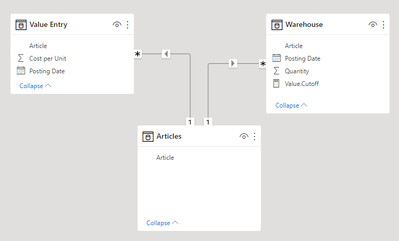cancel
Showing results for
Did you mean:

Fabric is Generally Available. Browse Fabric Presentations. Work towards your Fabric certification with the Cloud Skills Challenge.Regular Visitor

## Inventory average cost price per cut off period (selectedvalue/sumx/naturalinnerjoin/calcultables)

Hi all

I am trying to calculate the value of our inventory at cut off periods. The difficulty lies within the average cost price method which is recalculated at every new purchase. Our ERP system keeps track of every new average cost price (table1). In table2 i have all ins and outs of our warehouse.

I want to calculate the inventory value at cut off periods in the past based on a SelectedValue of a secondary date table (=SelectedDate). For example I select 30/09/2021 as cut off period. Here below you can find the steps (I think) I need to take to find my result. But I do not find a correct result.

=> Filter Table 1 on date <= SelectedDate and only keep the LASTDATE per article (these are marked in red)
=> Filter Table2 on date <= SelectedDate and summarize column quanitity per article
=> Multiply the unit cost from table 1 with the quantity of table 2 for each article

In the example from below this would get me
Article 1 => 12 * (50-10) = 480
Article 2 => 5 * (10-1) = 45

Table1 (Value Entry) = history of average cost prices

Article UnitCost  Date
1          10           01/09/2021
1          12           28/09/2021
1          11           15/10/2021
2          5             02/09/2021
2          6            10/10/2021

Table2 (Warehouse)= all stock movements ins and outs
Article Quantity Date
1          50             01/08/2021
1         -10            10/09/2021
2         10              31/08/2021
2         -1              01/09/2021
1        100            10/10/2021

I tried following code and this works quite okay on article level, but not on total level. I think the answer lies within a combination of calculatedtables and sumx/naturalinnerjoin but I do not see the light.

Value.Cutoff =
VAR SelectedDate = CALCULATE(SELECTEDVALUE(CutOffSelection[End of Month]))
VAR LastUnitCost = CALCULATE(sum('Value Entry'[Cost per Unit]),FILTER('Value Entry', 'Value Entry'[Posting Date] <=SelectedDate && MAX('Value Entry'[Posting Date])))
VAR LastUnitCostDate = calculate(MAX('Value Entry'[Posting Date]), FILTER('Value Entry','Value Entry'[Posting Date]<= SelectedDate))
Var costperunit = calculate(AVERAGE('Value Entry'[Cost per Unit]),FILTER('Value Entry','Value Entry'[Posting Date]=LastUnitCostDate))
VAR Quantity = calculate([W.Quantity],FILTER('Item Ledger Entry_Warehouse', 'Item Ledger Entry_Warehouse'[Posting Date]<=SelectedDate))
VAR Result= Quantity*costperunit

return Result

Thanks a lot!

Jordy

1 ACCEPTED SOLUTIONCommunity Support

Hi @DelVosa

Here is my solution. Hope it would be helpful.

First add a Dim Articles table to the model. Relate it to "Value Entry" and "Warehourse" tables on Article columns.Then create the following measure

``````Value.Cutoff =
VAR _selectedDate =
SELECTEDVALUE ( CutOffSelection[End of Month] )
VAR _table1 =
SUMMARIZE (
Articles,
Articles[Article],
"LastUnitCostDate",
CALCULATE (
MAX ( 'Value Entry'[Posting Date] ),
FILTER ( 'Value Entry', 'Value Entry'[Posting Date] <= _selectedDate )
),
"Quantity",
CALCULATE (
SUM ( Warehouse[Quantity] ),
FILTER ( Warehouse, Warehouse[Date] <= _selectedDate )
)
)
VAR _table2 =
_table1,
"LastUnitCost",
VAR _article = [Article]
VAR _lastDate = [LastUnitCostDate]
RETURN
CALCULATE (
MAX ( 'Value Entry'[Cost per Unit] ),
FILTER (
'Value Entry',
'Value Entry'[Article] = _article
&& 'Value Entry'[Posting Date] = _lastDate
)
)
)
RETURN
SUMX ( _table2, [Quantity] * [LastUnitCost] )
``````Sample file has been attached at bottom.

Best Regards,
Community Support Team _ Jing
If this post helps, please Accept it as Solution to help other members find it.Community Support

Hi @DelVosa

Here is my solution. Hope it would be helpful.

First add a Dim Articles table to the model. Relate it to "Value Entry" and "Warehourse" tables on Article columns.Then create the following measure

``````Value.Cutoff =
VAR _selectedDate =
SELECTEDVALUE ( CutOffSelection[End of Month] )
VAR _table1 =
SUMMARIZE (
Articles,
Articles[Article],
"LastUnitCostDate",
CALCULATE (
MAX ( 'Value Entry'[Posting Date] ),
FILTER ( 'Value Entry', 'Value Entry'[Posting Date] <= _selectedDate )
),
"Quantity",
CALCULATE (
SUM ( Warehouse[Quantity] ),
FILTER ( Warehouse, Warehouse[Date] <= _selectedDate )
)
)
VAR _table2 =
_table1,
"LastUnitCost",
VAR _article = [Article]
VAR _lastDate = [LastUnitCostDate]
RETURN
CALCULATE (
MAX ( 'Value Entry'[Cost per Unit] ),
FILTER (
'Value Entry',
'Value Entry'[Article] = _article
&& 'Value Entry'[Posting Date] = _lastDate
)
)
)
RETURN
SUMX ( _table2, [Quantity] * [LastUnitCost] )
``````Sample file has been attached at bottom.

Best Regards,
Community Support Team _ Jing
If this post helps, please Accept it as Solution to help other members find it.Announcements#### Power BI Monthly Update - November 2023

Check out the November 2023 Power BI update to learn about new features.#### Fabric Community News unified experience

Read the latest Fabric Community announcements, including updates on Power BI, Synapse, Data Factory and Data Activator.#### The largest Power BI and Fabric virtual conference

130+ sessions, 130+ speakers, Product managers, MVPs, and experts. All about Power BI and Fabric. Attend online or watch the recordings.Top Solution Authors
Top Kudoed Authors
Users online (3,671)